Related Articles

# How to display a PySpark DataFrame in table format ?

• Last Updated : 27 Jul, 2021

In this article, we are going to display the data of the PySpark dataframe in table format. We are going to use show() function and toPandas function to display the dataframe in the required format.

show(): Used to display the dataframe.

Attention geek! Strengthen your foundations with the Python Programming Foundation Course and learn the basics.

To begin with, your interview preparations Enhance your Data Structures concepts with the Python DS Course. And to begin with your Machine Learning Journey, join the Machine Learning - Basic Level Course

Syntax: dataframe.show( n, vertical = True, truncate = n)

where,

1. dataframe is the input dataframe
2. N is the number of rows to be displayed from the top ,if n is not specified it will print entire rows in the dataframe
3. vertical parameter specifies the data in the dataframe displayed in vertical format if it is true, otherwise it will display in horizontal format like a dataframe
4. truncate is a parameter us used to trim the values in the dataframe given as a number to trim

toPanads(): Pandas stand for a panel data structure which is used to represent data in a two-dimensional format like a table.

Syntax: dataframe.toPandas()

where, dataframe is the input dataframe

Let’s create a sample dataframe.

## Python3

 `# importing module``import` `pyspark` `# importing sparksession from``# pyspark.sql module``from` `pyspark.sql ``import` `SparkSession` `# creating sparksession and giving``# an app name``spark ``=` `SparkSession.builder.appName(``'sparkdf'``).getOrCreate()` `# list  of employee data with 5 row values``data ``=` `[[``"1"``, ``"sravan"``, ``"company 1"``],``        ``[``"2"``, ``"ojaswi"``, ``"company 2"``],``        ``[``"3"``, ``"bobby"``, ``"company 3"``],``        ``[``"4"``, ``"rohith"``, ``"company 2"``],``        ``[``"5"``, ``"gnanesh"``, ``"company 1"``]]` `# specify column names``columns ``=` `[``'Employee ID'``, ``'Employee NAME'``, ``'Company Name'``]` `# creating a dataframe from the lists of data``dataframe ``=` `spark.createDataFrame(data, columns)` `print``(dataframe)`

Output:

DataFrame[Employee ID: string, Employee NAME: string, Company Name: string]

Example 1: Using show() function without parameters. It will result in the entire dataframe as we have.

## Python3

 `# Display df using show()``dataframe.show()`

Output: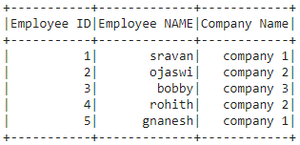Example 2: Using show() function with n as a parameter, which displays top n rows.

Syntax: DataFrame.show(n)

Where, n is a row

Code:

## Python3

 `# show() function to get 2 rows``dataframe.show(``2``)`

Output: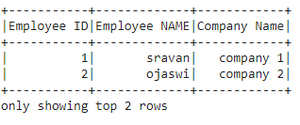Example 3:

Using show() function with vertical = True as parameter. Display the records in the dataframe vertically.

Syntax: DataFrame.show(vertical)

vertical can be either true and false.

Code:

## Python3

 `# display dataframe evrtically``dataframe.show(vertical ``=` `True``)`

Output: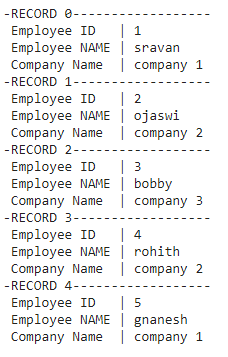Example 4: Using show() function with truncate as a parameter. Display first one letter in each value of all the columns

## Python3

 `# display dataframe with truncate``dataframe.show(truncate ``=` `1``)`

Output: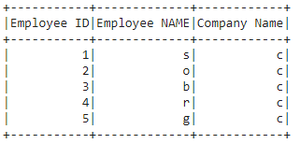Example 5: Using show() with all parameters.

## Python3

 `# display dataframe with all parameters``dataframe.show(n``=``3``,vertical``=``True``,truncate``=``2``)`

Output: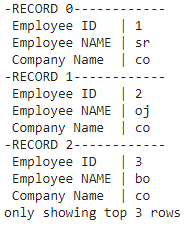Example 6: Using toPandas() method, which converts it to Pandas Dataframe which perfectly looks like a table.

## Python3

 `# display dataframe by using topandas() function``dataframe.toPandas()`

Output: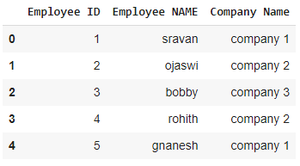My Personal Notes arrow_drop_up